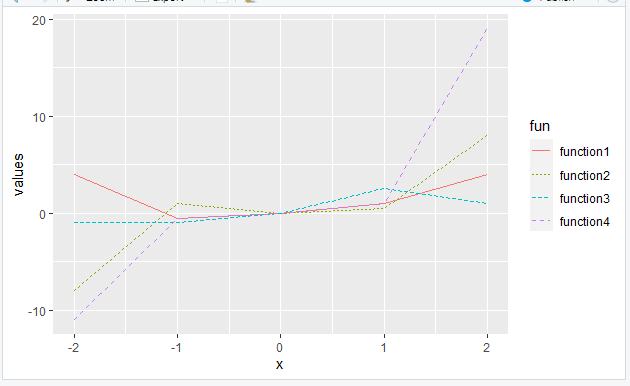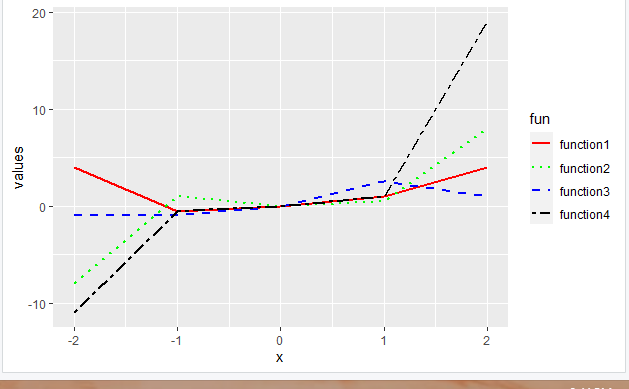# Control Line Color and Type in ggplot2 Plot Legend in R

• Last Updated : 21 Jul, 2021

In this article, we will see how to control line color and type in ggplot2 plot legend in the R programming language.

## Using Default Parameters

In this method, inbuilt attributes are passed to the function with appropriate values to generate the requirement. Thus, in order to change the color, col or color attribute has to be passed with the column name from the dataframe on the basis of which distinction has to be made.

Syntax: aes(col= column_name)

Similarly, to control line type, linetype attribute needs to be passed with the column name of the dataframe.

Syntax: aes(linetype= column_name )

Example: Controlling line color and type using default parameters

## R

 `library``(``"ggplot2"``)`` ` `function1 <- ``function``(x){x**2}``function2 <- ``function``(x){x**3}``function3 <- ``function``(x){x/2}``function4 <- ``function``(x){2*(x**3)+(x**2)-(x/2)}`` ` `df = ``data.frame``(x = -2:2,``              ``values = ``c``(``function1``(-2:2),``                       ``function2``(-2:2),``                       ``function3``(-2:2),``                       ``function4``(-2:2)),``              ``fun = ``rep``(``c``(``"function1"``,``"function2"``,``                        ``"function3"``,``"function4"``))``)`` ` `ggplot``(df, ``aes``(x, values, color = fun, ``               ``linetype = fun)) + ``geom_line``()`

Output:## Changing manually

Under this method, the color of the line plot is controlled using the scale_color_manual() function, which is passed with a vector of colors for each line.

Syntax: scale_color_manual(vector)

Similarly, linetype can be controlled using scale_linetype_manual() function, this also takes a vector as an input but the values passed are different linetypes for each line.

Syntax: scale_linetype_manual(vector)

Example: Controlling line color and type manually

## R

 `library``(``"ggplot2"``)``function1<- ``function``(x){x**2}``function2<-``function``(x){x**3}``function3<-``function``(x){x/2}``function4<-``function``(x){2*(x**3)+(x**2)-(x/2)}`` ` `df=``data.frame``(x=-2:2,``              ``values=``c``(``function1``(-2:2),``                       ``function2``(-2:2),``                       ``function3``(-2:2),``                       ``function4``(-2:2)),``              ``fun=``rep``(``c``(``"function1"``,``"function2"``,``"function3"``,``"function4"``))``)`` ` `ggplot``(df,``aes``(x,values, group=fun, color=fun, linetype=fun))+``geom_line``(size=1)+``  ``scale_linetype_manual``(values = ``c``(``"solid"``,``"dotted"``,``"dashed"``,``"twodash"``))+``  ``scale_color_manual``(values=``c``(``"red"``,``"green"``,``"blue"``,``"black"``))`

Output:My Personal Notes arrow_drop_up